ON INVERSE FUNCTIONS: INTRODUCTIONMany students in high school and in college have a difficult time with the concept of inverse functions: what they are, when they exist, how they are used, how to calculate them, how to graph them, and how to interpret their findings. Hopefully, this review will take some of the mystique away from these functions.

A Few Preliminaries:

First let’s review the definition of a function. Recall that first and foremost, a function is a rule. This rule links one set of numbers (called the domain) to a second set of numbers (called the range). The elements in the domain are independent. The value of the elements in the range depends on the value of the elements in the domain. All functions have a constraint on the rule: the rule can link a number in the domain to just one number in the range.

For example, suppose you are interviewing for a job at a telemarketing firm that pays \$10 per hour for as many hours as you wish to work, and the firm pays you at the end of each day. If you are trying to calculate your potential salary, the rule is Salary = \$10 times the number of hours you worked in a day. If you were to graph the rule, all the points on the graph would consists of pairs of numbers such as (number of hours worked, salary for hours worked). Can you see that the domain is restricted to the set of numbers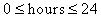? Since you can’t work less than zero hours or more than 24 hours in a day, the only numbers in the domain are the numbers between and including 0 to 24. How about the range? Can you see that the range is restricted to the set of numbers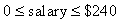? You cannot earn less than 0 dollars and you cannot earn more than \$240 dollars. The restriction on the rule that a number in the domain can be linked to just one number in the range means that if your pay is \$10 per hour, there is only one way to calculate your salary.

The inverse function is a kind of undoing function. Suppose you were told that Sally Perez earned \$140 dollars on Tuesday, could you work backwards and find the number of hours she worked? The inverse rule would be Salary / 10. If you divide Sally’s salary of \$140 by 10, you would know that she worked 14 hours on Tuesday. The first rule of Salary = \$10 x Number of Hours worked is a function. The undo rule of Number of Hours Worked = Salary / 10 is the inverse function.

The graphs of these two functions are related. For example, if the point (5 hours, \$50 salary) is located on the graph of the first function, you would know that the point (\$50 salary , 5 hours) would be located on the graph of the inverse function.

Suppose the rule for a function is "Multiply a number in the domain by 3 and add 7 to the product." We can translate this narrative rule to the mathematical model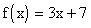. If we choose the number 10 from the domain, and we link it to a number in the range by following the function rule, the 10 will be linked to 37 because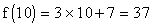. From this we know that the point (10, 37) is a point on the graph of the function f(x). We also know that the point (37, 10) is a point on the graph of the inverse function.

Can you find the rule that will undo the first rule? The first rule took 10 to 37, and now we need a rule to take 37 back to 10.

Let’s try. If we subtract 7 from the 37, we will get 30, and if we divide the 30 by 3, we will get a 10. Let’s try this with another pair of numbers. If 5 is in the domain of the function f(x), then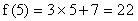is the function value and the (5, 22) is a point on the graph of the function. We know that (22, 5) is a point on the graph of the inverse function. The function rule took 5 to 22 and we want to find the inverse function rule that will take 22 back to 5. A shortcut for what we did with the first number is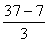. Let’s see if same steps that worked to undo the point (10, 37) will work with the point (5, 22). If it does, we have our rule. Subtract 7 from 22 to get 15 and divide the 15 by 3 to get 5. It works. A shortcut for what we did with this point is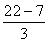. The inverse function rule is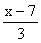.

We have to be able to denote the difference in notation between the function and its inverse. The standard notation is f(x) for the function and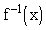for the inverse function. Do not confusewith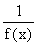. They are not the same thing.

The function rule is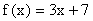. The inverse function rule is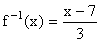. Let’s verify this rule with a few more points.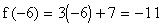and the point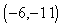is located on the graph of the function. Let’s verify that the inverse function will take the -11 back to the -6. It does because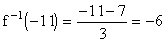and this means that the point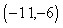is a point on the graph of the inverse function.

Study the following graphs of the function, the inverse function, and the line y = x. Can you see that if you mentally fold the page over the line y = x, that the graph of the function and the graph of the inverse function are superimposed? This means that the graphs of a function and its inverse are symmetric to each other with respect to the line y = x.

Find a point on the graph of the function below and see if you can find the undo point on the graph of the inverse function below.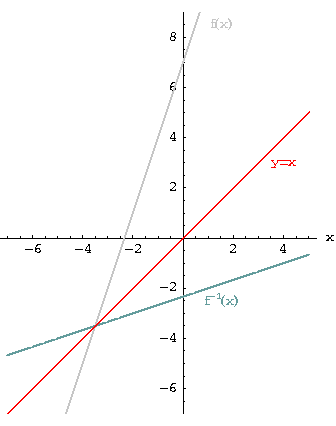Since the above rules were very simple, there was not much of an effort to figure out what the undo rule was. Calculating most inverse functions is not that simple. Before we really get into finding inverses, let’s review the concept of composition of functions because it is related to finding the inverse function.

Suppose that the rule for a function is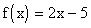. We know that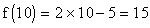or the rule links 10 to 15. Now suppose we have a second rule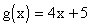. We can use this new rule to link the 15 to 65 because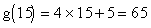. Since the first rule, f(x), operated on the 10, the 10 is in the domain of the function f(x). Since the f(x) rule linked the 10 to 15, the 15 is located in the range of f(x). The second rule, g(x), operated on the 15, therefore the 15 is also located in the domain of g(x). The g(x) rule links the 15 to 65, and therefore, the 65 is located in the range of g(x). Instead of going through all these steps, we can create a new function (a composition of f(x) and g(x)) that will take the 10 directly to the 65. Let’s call this new function h(x)..The new function is found as follows: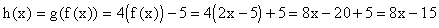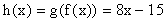Let’s check it out. If we are correct, the h(x) rule will link the 10 directly to 65.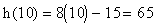Now let’s use this concept to calculate the inverse functions algebraically. Since the function f(x) links the number x to the number f(x), the inverse function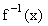links the f(x) back to the x. Another way of saying this is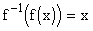. We can also say that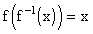.

Let’s see if this is true by composing the function in the first example,, with its inverse function,. Substitutein place of x in the formulaso that we have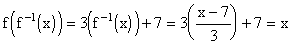. We have just shown that the function links x to f(x) and inverse functions links f(x) back to x.

Let’s see how we use the fact thatto find the inverse.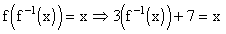• Solve forby first subtracting 7 from both sides of the equation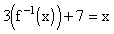to have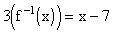.
• Now divide both sides ofby 3 and we have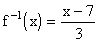.

Not all functions have inverses without some restriction on the domain.

Summary:

1. Only one-to-one functions have a unique inverse.
2. If the function is not one-to-one, the domain of the function must be restricted so that a portion of the graph is one-to-one. You can find a unique inverse over that portion of the restricted domain.
3. The domain of the function is equal to the range of the inverse. The range of the function is equal to the domain of the inverse.

If you would like to review some more examples on how to calculate an inverse function with no restriction on the domain, click on Example.

If you would like to review some more examples on how to calculate composition functions, click on composition.

If you would like to review explanations and examples on how to calculate the inverse function where the domain of the original function has to be restricted, click on restricted.[Next]
[Algebra] [Trigonometry] [Complex Variables]
[Calculus] [Differential Equations] [Matrix Algebra]S.O.S MATHematics home page

Do you need more help? Please post your question on our S.O.S. Mathematics CyberBoard.Author:Nancy Marcus